## 前言

• Python - 编程实践所使用的语言；
• Numpy - Python 的数值计算库。

## 矩阵

$\mathbf{A}_{2 \times 3}=\begin{bmatrix} 5 & 2 & 7 \\ 1 & 3 & 4 \end{bmatrix}$

$\mathbf{ B }_{ 4 \times 4 }=\begin{bmatrix} 5 & 2 & 7 & 6 \\ 1 & 3 & 4 & 2 \\ 7 & -1 & 9 & 0 \\ 8 & 2 & -2 & 3 \end{bmatrix}$

Python 的 Numpy 库提供了 ndarray 类用于存储高维数组及普通的数组运算，另外提供 matrix 类用来支持矩阵运算。使用 Python 创建矩阵很简单：

### 基本运算

#### 加

$\mathbf{A}+\mathbf{B} = \begin{bmatrix} 1 & 0 & 1 \\ 1 & 2 & 1 \\ 2 & 1 & 1 \end{bmatrix} + \begin{bmatrix} 2 & 1 & -1 \\ 0 & -1 & 2 \\ 2 & -1 & 0 \end{bmatrix} = \begin{bmatrix} 1+2 & 0+1 & 1+(-1) \\ 1+ 0 & 2+(-1) & 1+2 \\ 2+2 & 1+(-1) & 1+0 \end{bmatrix} = \begin{bmatrix} 3 & 1 & 0 \\ 1 & 1 & 3 \\ 4 & 0 & 1 \end{bmatrix}$

Python 示例：

#### 减

$\mathbf{A}-\mathbf{B}=\begin{bmatrix} 1 & 0 & 1 \\ 1 & 2 & 1 \\ 2 & 1 & 1 \end{bmatrix}-\begin{bmatrix} 2 & 1 & -1 \\ 0 & -1 & 2 \\ 2 & -1 & 0 \end{bmatrix}=\begin{bmatrix} 1-2 & 0-1 & 1-(-1) \\ 1-0 & 2-(-1) & 1-2 \\ 2-2 & 1-(-1) & 1-0 \end{bmatrix}=\begin{bmatrix} -1 & -1 & 2 \\ 1 & 3 & -1 \\ 0 & 2 & 1 \end{bmatrix}$

Python 示例：

#### 乘

\begin {aligned} \mathbf{A} \times \mathbf{B} &=\begin{bmatrix} 1 & 0 & 1 \\ 1 & 2 & 1 \\ 2 & 1 & 1 \end{bmatrix}\times \begin{bmatrix} 2 & 1 & -1 \\ 0 & -1 & 2 \\ 2 & -1 & 0 \end{bmatrix} \\\ &=\begin{bmatrix} 1\cdot 2+0\cdot 0+1\cdot 2 & 1\cdot 1+0\cdot (-1)+1\cdot (-1) & 1\cdot (-1)+0\cdot 2+1\cdot 0 \\ 1\cdot 2+2\cdot 0+1\cdot 2 & 1\cdot 1+2\cdot (-1)+1\cdot (-1) & 1\cdot (-1)+2\cdot 2+1\cdot 0 \\ 2\cdot 2+1\cdot 0+1\cdot 2 & 2\cdot 1+1\cdot (-1)+1\cdot (-1) & 2\cdot (-1)+1\cdot 2+1\cdot 0 \end{bmatrix}\\\ &=\begin{bmatrix} 4 & 0 & -1 \\ 4 & -2 & 3 \\ 6 & 0 & 0 \end{bmatrix} \end {aligned}

$\mathbf{C}\times \mathbf{D} = \begin{bmatrix} 5 & 7 & 2 \\ 4 & 3 & 1 \end{bmatrix}\times \begin{bmatrix} 1 \\ 5 \\ 6 \end{bmatrix} =\begin{bmatrix} 5 \cdot 1+ 7 \cdot 5+ 2\cdot 6 \\ 4\cdot 1+3\cdot 5+1\cdot 6 \end{bmatrix} =\begin{bmatrix} 52 \\ 25 \end{bmatrix}$

1. 单元矩阵 $\mathbf{I}$ 。它的特点是行数列数相等，且在对角线上值为 1，其他地方值为 0 。它的一个特性是与其他矩阵相乘都等于那个矩阵本身。一个 $3\times 3$ 的单元矩阵示例：$\mathbf{I}_{3 \times 3} = \begin{bmatrix} 1 & 0 & 0 \\ 0 & 1 & 0 \\ 0 & 0 & 1 \end{bmatrix}$
2. 零矩阵。顾名思义就是全部元素都是 0 的矩阵。零矩阵乘以任何矩阵都为零矩阵，与任何矩阵相加都等于那个矩阵。

Python 示例：

#### 除（求逆）

##### 伴随阵法

$\mathbf{A}^{-1}=\frac{1}{|\mathbf{A}|}\begin{bmatrix}A_{11} & A_{21} & \ldots & A_{n1} \\ A_{12} & A_{22} & \ldots & A_{n2} \\ \ldots & \ldots & \ldots & \ldots \\ A_{1n} & A_{2n} & \ldots & A_{nn} \end{bmatrix}$

$\mathbf{M}^{-1}=\frac{1}{|\mathbf{M}|}\begin{bmatrix} d & -b \\ -c & a \end{bmatrix}$

，其中 $|\mathbf{M}|$ 称为矩阵 $\mathbf{M}$ 的行列式：

$|\mathbf{M}| = ad - bc$

，而 $\begin{bmatrix} d & -b \\ -c & a \end{bmatrix}$ 就是矩阵 $\mathbf{M}$ 的伴随矩阵。

$|\mathbf{A}|=5\cdot 2-7\cdot 3=-11$

，则

$\mathbf{A}^{ -1 }=\frac { 1 }{ -11 } \begin{bmatrix} 2 & -7 \\ -3 & 5 \end{bmatrix}=\begin{bmatrix} -\frac { 2 }{ 11 } & \frac { 7 }{ 11 } \\ \frac { 3 }{ 11 } & -\frac { 5 }{ 11 } \end{bmatrix}$

$\mathbf{A}\times \mathbf{A}^{ -1 }=\begin{bmatrix} 5 & 7 \\ 3 & 2 \end{bmatrix}\times \begin{bmatrix} -\frac { 2 }{ 11 } & \frac { 7 }{ 11 } \\ \frac { 3 }{ 11 } & -\frac { 2 }{ 11 } \end{bmatrix}=\begin{bmatrix} 5\cdot \left( -\frac { 2 }{ 11 } \right) +7\cdot \frac { 3 }{ 11 } & 5\cdot \frac { 7 }{ 11 } +\left( -7\cdot \frac { 5 }{ 11 } \right) \\ 3\cdot \left( -\frac { 2 }{ 11 } \right) +2\cdot \frac { 3 }{ 11 } & 3\cdot \frac { 7 }{ 11 } +2\cdot \left( -\frac { 5 }{ 11 } \right) \end{bmatrix}=\begin{bmatrix} 1 & 0 \\ 0 & 1 \end{bmatrix} = \mathbf{I}$

##### 初等变换法

1. 对调两行；
2. 以数 $k \ne 0$ 乘某一行的所有元素；
3. 把某一行所有元素的 $k$ 倍加到另一行对应的元素上去。

\begin{aligned} \begin{bmatrix}\mathbf{A} \mathbf{I}\end{bmatrix} & \rightarrow \begin{bmatrix} 1 & 0 & 1 & 1 & 0 & 0 \\ 1 & 2 & 1 & 0 & 1 & 0 \\ 2 & 1 & 1 & 0 & 0 & 1 \end{bmatrix} \rightarrow \begin{bmatrix} 1 & 0 & 1 & 1 & 0 & 0 \\ 0 & 2 & 0 & -1 & 1 & 0 \\ 2 & 1 & 1 & 0 & 0 & 1 \end{bmatrix} \rightarrow \begin{bmatrix} 1 & 0 & 1 & 1 & 0 & 0 \\ 0 & 1 & 0 & -0.5 & 0.5 & 0 \\ 2 & 1 & 1 & 0 & 0 & 1 \end{bmatrix}\\ & \rightarrow \begin{bmatrix} 1 & 0 & 1 & 1 & 0 & 0 \\ 0 & 1 & 0 & -0.5 & 0.5 & 0 \\ 1 & 1 & 0 & -1 & 0 & 1 \end{bmatrix} \rightarrow \begin{bmatrix} 1 & 0 & 1 & 1 & 0 & 0 \\ 0 & 1 & 0 & -0.5 & 0.5 & 0 \\ 1 & 0 & 0 & -0.5 & -0.5 & 1 \end{bmatrix} \rightarrow \begin{bmatrix} 0 & 0 & 1 & 1.5 & 0.5 & -1 \\ 0 & 1 & 0 & 0 & 0.5 & 0 \\ 1 & 0 & 0 & -0.5 & -0.5 & 1 \end{bmatrix}\\ &\rightarrow \begin{bmatrix} 1 & 0 & 0 & -0.5 & -0.5 & 1 \\ 0 & 1 & 0 & -0.5 & 0.5 & 0 \\ 0 & 0 & 1 & 1.5 & 0.5 & -1 \end{bmatrix} \end{aligned}

$\mathbf{A}^{-1}=\begin{bmatrix}-0.5 & -0.5 & 1 \\ -0.5 & 0.5 & 0 \\ 1.5 & 0.5 & -1\end{bmatrix}$

Python 求逆示例：

Python 示例：

Python 示例：

### 应用举例

#### 求解方程组

$\left\{ \begin{eqnarray} 3x+2y & = & 7 \\ -x+y & = & 1 \end{eqnarray} \right.$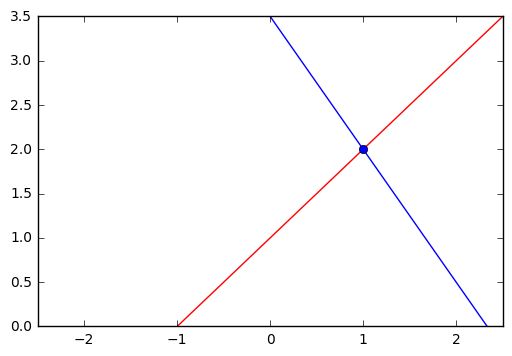$\begin{bmatrix} 3 & 2 \\ -1 & 1 \end{bmatrix} \begin{bmatrix} x \\ y \end{bmatrix} = \begin{bmatrix} 7\\ 1 \end{bmatrix}$

\begin{aligned} A^{-1}A \begin{bmatrix} x \\ y \end{bmatrix} &= A^{-1} \begin{bmatrix} 7\\ 1 \end{bmatrix}\\\ &= \frac{1}{|A|}\begin{bmatrix}1 & -2 \\ 1 & 3\end{bmatrix} \begin{bmatrix} 7\\ 1 \end{bmatrix}\\\ &= \frac{1}{5}\begin{bmatrix}1 & -2 \\ 1 & 3\end{bmatrix} \begin{bmatrix} 7\\ 1 \end{bmatrix}\\\ &= \frac{1}{5}\begin{bmatrix}5 \\ 10\end{bmatrix} \end{aligned}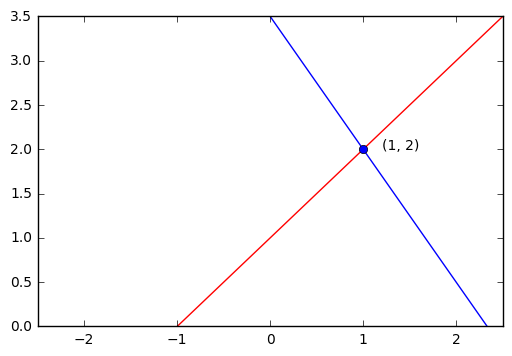#### 求向量组合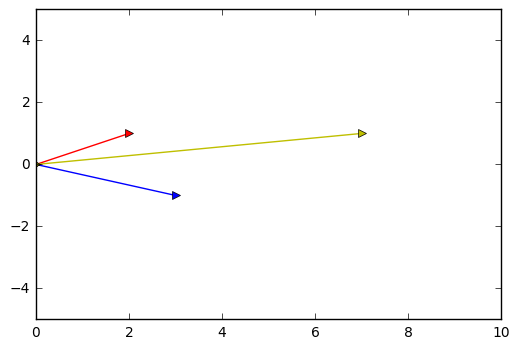$\begin{bmatrix} 3 \\ -1 \end{bmatrix} x + \begin{bmatrix} 2 \\ 1 \end{bmatrix} y = \begin{bmatrix} 7\\ 1 \end{bmatrix}$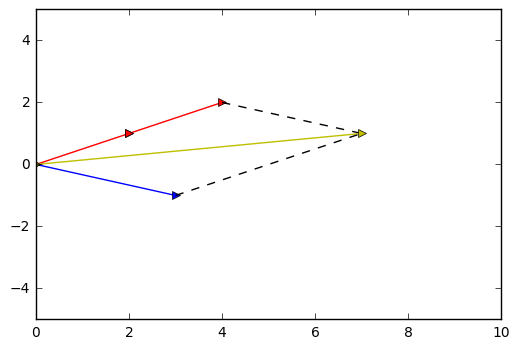## 向量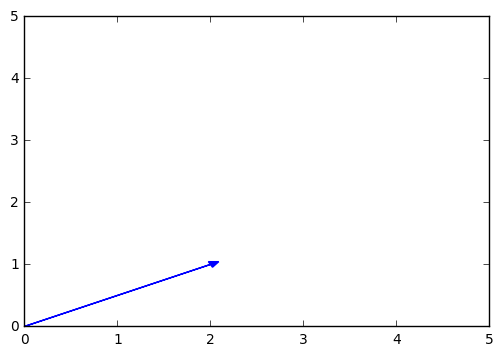### 基本运算

#### 加

$\mathbf{a} + \mathbf{b} = \begin{bmatrix} a_1 + b_1 \\ a_2 + b_2 \\ \ldots \\ a_n + b_n \end{bmatrix}$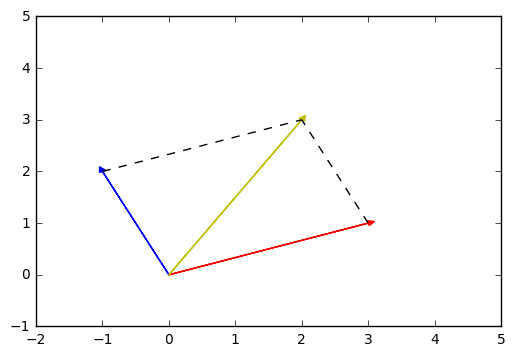#### 减

$\mathbf{a} - \mathbf{b} = \begin{bmatrix} a_1 - b_1 \\ a_2 - b_2 \\ \ldots \\ a_n - b_n \end{bmatrix}$

#### 乘

##### 标量乘向量

$c \cdot \mathbf{a} = \begin{bmatrix} c \cdot a_1 \\ c \cdot a_2 \\ \ldots \\ c \cdot a_n \end{bmatrix} = \begin{bmatrix} a_1 \cdot c \\ a_2 \cdot c \\ \ldots \\ a_n \cdot c \end{bmatrix}$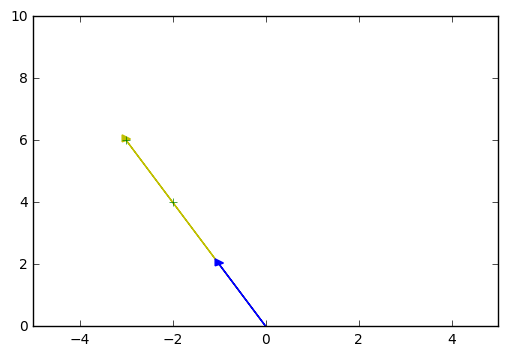Python 实现：

##### 向量点积

$\vec{\mathbf{a}}\cdot \vec{\mathbf{b}} = \begin{bmatrix} a_1 \\ a_2 \\ \ldots \\ a_n\end{bmatrix} \cdot \begin{bmatrix} b_1 \\ b_2 \\ \ldots \\ b_n \end{bmatrix} = a_{1}b_{1} + a_{2}b_{2} + \ldots + a_{n}b_{n}$

$\begin{bmatrix} 3 \\ 5 \\ 2 \end{bmatrix} \cdot \begin{bmatrix} 1 \\ 4 \\ 7 \end{bmatrix} = 3 \cdot 1 + 5 \cdot 4 + 2 \cdot 7 = 37$

Python 示例：

eq: 1 »
$\left\| \vec{\mathbf{v}} \right\| = \sqrt{v_{1}^{2} + v_{2}^{2} + \ldots + v_{n}^{2}} = \sqrt{\vec{\mathbf{v}} \cdot \vec{\mathbf{v}}}$

• 对两个非 0 向量 $\vec{\mathbf{x}}, \vec{\mathbf{y}} \in \mathbb{R}^{n}$，$|\vec{\mathbf{x}} \cdot \vec{\mathbf{y}}| \le \left\|\vec{\mathbf{x}}\right\|\left\|\vec{\mathbf{y}}\right\|$。
• 当且仅当 $\vec{\mathbf{x}} = c\vec{\mathbf{y}}$ 时，等式成立。

\begin{align} \left\|\vec{\mathbf{x}} + \vec{\mathbf{y}}\right\|^2 & = (\vec{\mathbf{x}} + \vec{\mathbf{y}})\cdot (\vec{\mathbf{x}} + \vec{\mathbf{y}}) \\\ & = \left\|\vec{\mathbf{x}}\right\|^2 + 2\vec{\mathbf{x}}\vec{\mathbf{y}} + \left\|\vec{\mathbf{y}}\right\|^2 \\\ & \le \left\|\vec{\mathbf{x}}\right\|^2 + 2\left\|\vec{\mathbf{x}}\right\|\left\|\vec{\mathbf{y}}\right\| + \left\|\vec{\mathbf{y}}\right\|^2 \end{align}

$\left\|\vec{\mathbf{x}} + \vec{\mathbf{y}}\right\|^2 \le (\left\|\vec{\mathbf{x}}\right\| + \left\|\vec{\mathbf{y}}\right\|)^2$

$\left\|\vec{\mathbf{x}} + \vec{\mathbf{y}}\right\| \le \left\|\vec{\mathbf{x}}\right\| + \left\|\vec{\mathbf{y}}\right\|$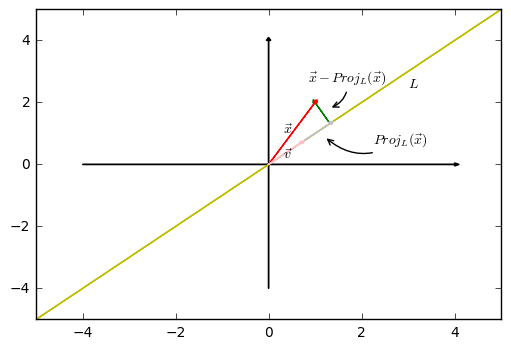\begin{align} (\vec{\mathbf{x}}-\underbrace { c\vec{\mathbf{v}}}_{ Proj_L({\vec{\mathbf{x}}}) } )\cdot \vec{\mathbf{v}} &= 0 \\\ \vec{\mathbf{x}}\cdot \vec{\mathbf{v}} -c\vec{\mathbf{v}}\cdot \vec{\mathbf{v}} &= 0\\\ c\cdot \vec{\mathbf{v}} \cdot \vec{\mathbf{v}} &= \vec{\mathbf{x}}\cdot \vec{\mathbf{v}}\\\ c &= \frac{\vec{\mathbf{x}}\cdot \vec{\mathbf{v}}}{\vec{\mathbf{v}}\cdot \vec{\mathbf{v}}} \end{align}

$Proj_L({\vec{\mathbf{x}}}) = c\vec{\mathbf{v}} = (\frac{\vec{\mathbf{x}}\cdot \vec{\mathbf{v}}}{\vec{\mathbf{v}}\cdot \vec{\mathbf{v}}})\vec{\mathbf{v}}$

$Proj_L({\vec{\mathbf{a}}}) = c\vec{\mathbf{b}} = (\frac{\vec{\mathbf{a}}\cdot \vec{\mathbf{b}}}{\vec{\mathbf{b}}\cdot \vec{\mathbf{b}}})\vec{\mathbf{b}} = \frac{3}{2}\vec{\mathbf{b}}$

Python 示例：

##### 向量外积

$\mathbb{R}^{2}$ 的向量外积：

$\begin{bmatrix} a_1 \\ a_2\end{bmatrix} \times \begin{bmatrix} b_1 \\ b_2 \end{bmatrix} = \begin{bmatrix} a_1 \cdot b_2 - a_2 \cdot b_1\end{bmatrix}$

$\begin{bmatrix} 1 \\ 2 \end{bmatrix} \times \begin{bmatrix} 3 \\ 4 \end{bmatrix} = \begin{bmatrix} 1 \cdot 4 - 3 \cdot 2 \end{bmatrix} = \begin{bmatrix}-2\end{bmatrix}$

$\mathbb{R}^{3}$ 的向量外积：

$\begin{bmatrix} a_1 \\ a_2 \\ a_3\end{bmatrix} \times \begin{bmatrix} b_1 \\ b_2 \\ b_3 \end{bmatrix} = \begin{bmatrix} a_2 \cdot b_3 - a_3 \cdot b_2 \\ a_3 \cdot b_1 - a_1 \cdot b_3 \\ a_1 \cdot b_2 - a_2 \cdot b_1\end{bmatrix}$

$\begin{bmatrix} 3 \\ 5 \\ 2 \end{bmatrix} \times \begin{bmatrix} 1 \\ 4 \\ 7 \end{bmatrix} = \begin{bmatrix} 5 \cdot 7 - 2 \cdot 4 \\ 2 \cdot 1 - 3 \cdot 7 \\ 3 \cdot 4 - 5 \cdot 1\end{bmatrix} = \begin{bmatrix} 27 \\ -19 \\ 7\end{bmatrix}$

Python 示例：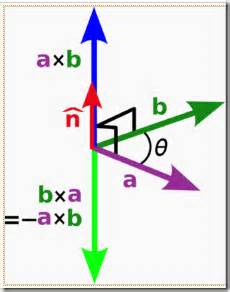##### 矩阵向量积

$\underset{m\times n}{A}\vec{\mathbf{x}}=\begin{bmatrix}a_{11} & a_{12} & \ldots & a_{1n} \\ a_{21} & a_{22} & \ldots & a_{2n} \\ \ldots \\ a_{m1} & a_{m2} & \ldots & a_{mn}\end{bmatrix}\begin{bmatrix}x_1 \\ x_2 \\ \ldots \\ x_n \end{bmatrix} = \begin{bmatrix}a_{11}x_1 + a_{12}x_2 + \ldots + a_{1n}x_n \\ a_{21}x_1 + a_{22}x_2 + \ldots + a_{2n}x_n \\ \ldots \\ a_{m1}x_1 + a_{m2}x_2 + \ldots + a_{mn}x_n \\ \end{bmatrix}$

$\begin{bmatrix}4\cdot 5 + 3\cdot 2 + 1\cdot 7 \\ 1 \cdot 5 + 2 \cdot 2 + 5 \cdot 7\end{bmatrix} = \begin{bmatrix}33 \\ 44\end{bmatrix}$

Python 示例：

$\underset { m\times n }{ \mathbf{A} } \vec { \mathbf{x} } =\begin{bmatrix} \underbrace { \begin{bmatrix} a_{ 11 } \\ a_{ 21 } \\ \ldots \\ a_{ m1 } \end{bmatrix} }_{ \vec { \mathbf{ V }_{ 1 } } } & \underbrace { \begin{bmatrix} a_{ 12 } \\ a_{ 22 } \\\ldots \\ a_{ m2 } \end{bmatrix} }_{ \vec { \mathbf{ V_{ 2 } } } } & \ldots & \underbrace { \begin{bmatrix} a_{ 1n } \\ a_{ 2n } \\ \ldots \\ a_{ mn } \end{bmatrix} }_{ \vec { \mathbf{ V_{ n } } } } \end{bmatrix}\begin{bmatrix} x_{ 1 } \\ x_{ 2 } \\ \ldots \\ x_{ n } \end{bmatrix}=x_1\vec{\mathbf{V}_1}+x_2\vec{\mathbf{V}_2}+\ldots+x_n\vec{\mathbf{V}_n}$

Python 示例：

### 线性无关

#### 张成空间

$c_1 \begin{bmatrix}2 \\ 1\end{bmatrix} + c_2 \begin{bmatrix} 0 \\ 3 \end{bmatrix} = \begin{bmatrix} x \\ y \end{bmatrix}$

\left\{ \begin{align} c_1 \cdot 2 & + c_2 \cdot 0 &= x\\\ c_1 \cdot 1 & + c_2 \cdot 3 &= y \end{align} \right.

\left\{ \begin{align} c_1 &= \frac{x}{2}\\ c_2 &= \frac{y}{3} - \frac{x}{6} \end{align} \right.

#### 判断是否线性相关

$c_1 \begin{bmatrix}2 \\ 1\end{bmatrix} + c_2 \begin{bmatrix}3 \\ 2\end{bmatrix} = \begin{bmatrix}0 \\ 0\end{bmatrix}$

## 线性代数进阶

### 阶梯形矩阵

#### 阶梯形矩阵

1. 若有零行（元素全为0的行），则零行应在最下方；
2. 非零首元（即非零行的第一个不为零的元素）的列标号随行标号的增加而严格递增。

$\begin{bmatrix} 2 & 0 & 2 & 1 \\ 0 & 5 & 2 & -2 \\ 0 & 0 & 3 & 2 \\ 0 & 0 & 0 & 0 \end{bmatrix}$

#### 行简化阶梯形矩阵

1. 它是阶梯形矩阵；
2. 非零首元所在的列除了非零首元外，其余元素全为0。

$\begin{bmatrix} 2 & 0 & 2 & 1 \\ 0 & 5 & 2 & -2 \\ 0 & 0 & 3 & 2 \\ 0 & 0 & 0 & 0 \end{bmatrix}$

1. 它是行简化阶梯形矩阵；
2. 非零首元都为1

#### 将矩阵化简成行最简阶梯形

$\begin{bmatrix} 1 & 2 & 1 & 1 & 7\\ 1 & 2 & 2 & -1 & 12\\ 2 & 4 & 0 & 6 & 4 \end{bmatrix}$

，使用初等变换可以将这个矩阵转换成如下的形式：

$\begin{bmatrix} 1 & 2 & 1 & 1 & 7\\ 1 & 2 & 2 & -1 & 12\\ 2 & 4 & 0 & 6 & 4 \end{bmatrix} \rightarrow \begin{bmatrix} 1 & 2 & 1 & 1 & 7\\ 0 & 0 & 1 & -2 & 5\\ 2 & 4 & 0 & 6 & 4 \end{bmatrix} \rightarrow \begin{bmatrix} 1 & 2 & 1 & 1 & 7\\ 0 & 0 & 1 & -2 & 5\\ 0 & 0 & -2 & 4 & -10 \end{bmatrix} \rightarrow \begin{bmatrix} 1 & 2 & 1 & 1 & 7\\ 0 & 0 & 1 & -2 & 5\\ 0 & 0 & 0 & 0 & 0 \end{bmatrix} \rightarrow \begin{bmatrix} 1 & 2 & 0 & 3 & 2\\ 0 & 0 & 1 & -2 & 5\\ 0 & 0 & 0 & 0 & 0 \end{bmatrix}$

$\left\{ \begin{eqnarray} x_1 + 2x_2 + x_3 + x_4 &=& 7 \\\ x_1 + 2x_2 + 2x_3 - x_4 &=& 12 \\\ 2x_1 + 4x_2 + 6x_4 &=& 4 \end{eqnarray} \right.$

$\begin{bmatrix} 1 & 2 & 1 & 1 & 7\\ 1 & 2 & 2 & -1 & 12\\ 2 & 4 & 0 & 6 & 4 \end{bmatrix}$

$\begin{bmatrix} 1 & 2 & 0 & 3 & 2\\ 0 & 0 & 1 & -2 & 5\\ 0 & 0 & 0 & 0 & 0 \end{bmatrix}$

$\left\{ \begin{eqnarray} x_1 &=& 2 - 2x_2 - 3x_4 \\\ x_3 &=& 5 + 2x_4 \end{eqnarray} \right.$

$\begin{bmatrix} x_{ 1 } \\ x_{ 2 } \\ x_{ 3 } \\ x_{ 4 } \end{bmatrix}=\begin{bmatrix} 2 \\ 0 \\ 5 \\ 0 \end{bmatrix}+x_{ 2 }\underbrace { \begin{bmatrix} -2 \\ 1 \\ 0 \\ 0 \end{bmatrix} }_{ \vec{\mathbf{a}} } +x_{ 4 }\underbrace{\begin{bmatrix} -3 \\ 0 \\ 2 \\ 1 \end{bmatrix}}_{\vec{\mathbf{b}}}$

### 线性子空间

1. 零向量 0 在 $\mathbf{W}$ 中。
2. 加法封闭：如果 $\vec{\mathbf{u}}$ 和 $\vec{\mathbf{v}}$ 是 $\mathbf{W}$ 的元素，则向量和 $\mathbf{\vec{\mathbf{u}}+\vec{\mathbf{v}}}$ 是 $\mathbf{W}$ 的元素。
3. 标量乘法封闭：如果 $\vec{\mathbf{u}}$ 是 $\mathbf{W}$ 的元素而 $c$ 是标量，则标量积 $c\vec{\mathbf{u}}$ 是 $\mathbf{W}$ 的元素。

#### 零空间

$\begin{bmatrix} 1 & 1 & 1 & 1 \\ 1 & 2 & 3 & 4 \\ 4 & 3 & 2 & 1 \end{bmatrix} \begin{bmatrix} x_1 \\ x_2 \\ x_3 \\ x_4 \end{bmatrix} = \begin{bmatrix} 0 \\ 0 \\ 0 \end{bmatrix}$

$\left\{ \begin{eqnarray} x_1 + x_2 + x_3 + x_4 &=& 0 \\\ x_1 + 2x_2 + 3x_3 + 4x_4 &=& 0 \\\ 4x_1 + 3x_2 + 2x_4 + x_4 &=& 0 \end{eqnarray} \right.$

$\begin{bmatrix} 1 & 1 & 1 & 1 & 0 \\ 1 & 2 & 3 & 4 & 0 \\ 4 & 3 & 2 & 1 & 0 \end{bmatrix}$

$\begin{bmatrix} 1 & 0 & -1 & -2 & 0 \\ 0 & 1 & 2 & 3 & 0 \\ 0 & 0 & 0 & 0 & 0 \end{bmatrix}$

$\begin{bmatrix} x_{ 1 } \\ x_{ 2 } \\ x_{ 3 } \\ x_{ 4 } \end{bmatrix}=x_{ 3 }\underbrace { \begin{bmatrix} 1 \\ -2 \\ 1 \\ 0 \end{bmatrix} }_{ \vec{\mathbf{a}} } +x_{ 4 }\underbrace{\begin{bmatrix} 2 \\ -3 \\ 0 \\ 1 \end{bmatrix}}_{\vec{\mathbf{b}}}$

$N(\mathbf{A}) = span\left(\begin{bmatrix} 1 \\ -2 \\ 1 \\ 0 \end{bmatrix} \begin{bmatrix} 2 \\ -3 \\ 0 \\ 1 \end{bmatrix}\right)$

#### 列空间

$C(\mathbf{A}) = span(\vec{v_1}, \vec{v_2}, \ldots, \vec{v_n})$

$\begin{bmatrix} 1 & 0 & -1 & -2 \\ 0 & 1 & 2 & 3 \\ 0 & 0 & 0 & 0 \end{bmatrix}$

import numpy as np
a = np.matrix('1 1 1 1;1 2 3 4; 0 0 1 0')
print a.ndim  # 2（维度）
print np.rank(a)  # 2（a.ndim 的别名，已经过时）
print np.linalg.matrix_rank(a)  # 3（秩）


#### 左零空间

$N(\mathbf{A}^T) = \left\{ \vec{\mathbf{x}} | \mathbf{A}^{T} \vec{\mathbf{x}} = \vec{\mathbf{0}} \right\} = \left\{ \vec{\mathbf{x}} | \vec{\mathbf{x}}^{T} \mathbf{A} = \vec{\mathbf{0}}^{T} \right\}$

### 最小二乘逼近

$\underset{n\times k}{\mathbf{A}}\vec{\mathbf{x}} = \vec{\mathbf{b}}$

$\vec{a_1}\vec{\mathbf{x}} + \vec{a_2}\vec{\mathbf{x}} + \ldots + \vec{a_k}\vec{\mathbf{x}} = \vec{\mathbf{b}}$

$\left\| \begin{bmatrix} \vec{b_1}-\vec{v_1}\\ \vec{b_2}-\vec{v_2}\\ \ldots\\ \vec{b_n}-\vec{v_n}\\ \end{bmatrix} \right\|^2 = (b_1-v_1)^2 + (b_2-v_2)^2 + \ldots + (b_n-v_n)^2$

$\mathbf{A}\vec{\mathbf{x}^*} = Proj_{C(\mathbf{A})}\vec{\mathbf{b}}$

$\mathbf{A}^T\mathbf{A}\vec{\mathbf{x}^*} = \mathbf{A}^T\vec{\mathbf{b}}$

#### 实例1：求解方程

$\left\{ \begin{eqnarray} x + y &=& 3 \\\ x - y &=& -2 \\\ y &=& 1 \end{eqnarray} \right.$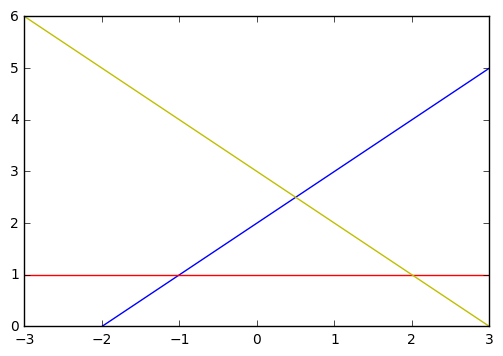$\underbrace{ \begin{bmatrix} 1 & 1 \\ 1 & -1 \\ 0 & 1 \end{bmatrix} }_{\mathbf{A}} \underbrace{ \begin{bmatrix} x \\ y \end{bmatrix} }_{\vec{\mathbf{x}}} = \underbrace{ \begin{bmatrix} 3 \\ -2 \\ 1 \end{bmatrix} }_{\vec{\mathbf{b}}}$

\begin{align} \begin{bmatrix} 1 & 1 & 0 \\ 1 & -1 & 1\\ \end{bmatrix} \begin{bmatrix} 1 & 1 \\ 1 & -1 \\ 0 & 1 \end{bmatrix} \begin{bmatrix} x^* \\ y^* \end{bmatrix} & = \begin{bmatrix} 1 & 1 & 0 \\ 1 & -1 & 1\\ \end{bmatrix} \begin{bmatrix} 3 \\ -2 \\ 1 \end{bmatrix} \\\ \begin{bmatrix} 2 & 0 \\ 0 & 3 \end{bmatrix} \begin{bmatrix} x^* \\ y^* \end{bmatrix} & = \begin{bmatrix} 1 \\ 6 \end{bmatrix} \end{align}

$\begin{bmatrix} x^* \\ y^* \end{bmatrix} = \begin{bmatrix}\frac{1}{2} & 0 \\ 0 & \frac{1}{3}\end{bmatrix} \begin{bmatrix} 1 \\ 6 \end{bmatrix} = \begin{bmatrix} \frac{1}{2} \\ 2 \end{bmatrix}$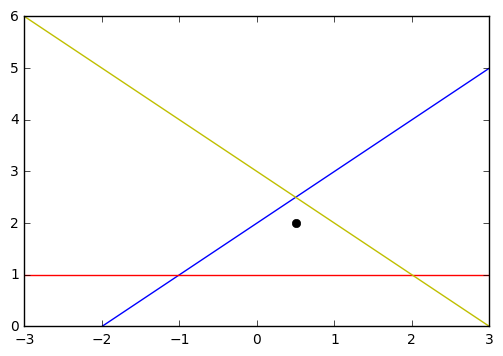numpy.linalg.lstsq 的返回包括四个部分：

1. 最小二乘逼近解。如果 b 是二维的，那么这个逼近的结果有多个列，每一列是一个逼近解。对于上例，逼近解就是 $\begin{bmatrix}0.5 \\ 2 \end{bmatrix}$ 。
2. 残差。即每一个 b - a*x 的长度的和。对于上例，残差是 1.5 。
3. 矩阵 a 的秩。对于上例，矩阵 a 的秩为 2 。
4. 矩阵 a 的奇异值。对于上例，矩阵 a 的奇异值为 $\begin{bmatrix}1.73205081 \\ 1.41421356\end{bmatrix}$

#### 实例2：线性回归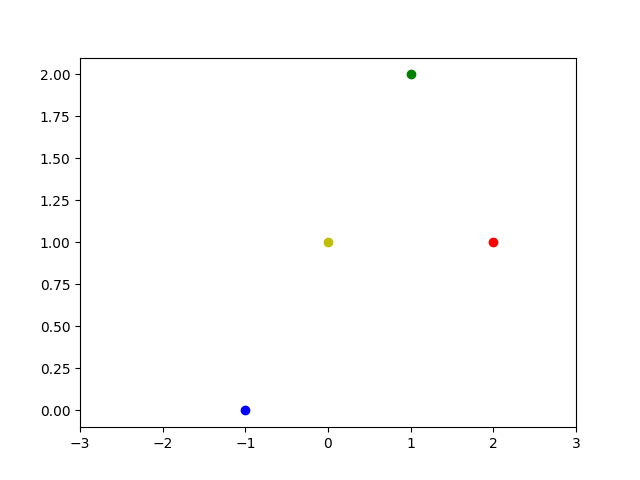$\left\{ \begin{eqnarray} f(-1) &= -m + b = 0\\\ f(0) &= 0 + b = 1\\\ f(1) &= m + b = 2\\\ f(2) &= 2m + b = 1 \end{eqnarray} \right.$

$\underbrace{ \begin{bmatrix} -1 & 1 \\ 0 & 1 \\ 1 & 1 \\ 2 & 1 \end{bmatrix} }_{\mathbf{A}} \underbrace{ \begin{bmatrix} m\\ b \end{bmatrix} }_{\vec{\mathbf{x}}} = \underbrace{ \begin{bmatrix} 0\\ 1\\ 2\\ 1 \end{bmatrix} }_{\vec{\mathbf{b}}}$

\begin{align} \begin{bmatrix} -1 & 0 & 1 & 2 \\ 1 & 1 & 1 & 1 \end{bmatrix} \begin{bmatrix} -1 & 1 \\ 0 & 1 \\ 1 & 1 \\ 2 & 1 \end{bmatrix} \begin{bmatrix} m^*\\ b^* \end{bmatrix} &= \begin{bmatrix} -1 & 0 & 1 & 2 \\ 1 & 1 & 1 & 1 \end{bmatrix} \begin{bmatrix} 0\\ 1\\ 2\\ 1 \end{bmatrix}\\\ \begin{bmatrix} 6 & 2 \\ 2 & 4 \end{bmatrix} \begin{bmatrix} m^*\\ b^* \end{bmatrix} &= \begin{bmatrix} 4\\ 4 \end{bmatrix} \end{align}

$\begin{bmatrix}m^*\\b^*\end{bmatrix} = \frac{1}{20}\begin{bmatrix}4 & -2\\-2 & 6\end{bmatrix}\begin{bmatrix}4 \\ 4\end{bmatrix} = \frac{1}{20}\begin{bmatrix}8 \\ 16\end{bmatrix} = \begin{bmatrix}\frac{2}{5} \\ \frac{4}{5}\end{bmatrix}$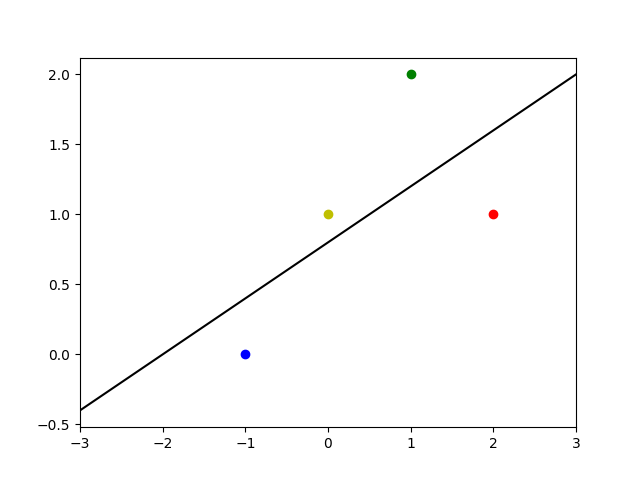Python 示例如下：

### 特征向量

“特征”在模式识别和图像处理中是非常常见的一个词汇。我们要认识和描绘一件事物，首先得找出这个事物的特征。同样的道理，要让计算机识别一件事物，首先就要让计算机学会理解或者抽象出事物的特征。什么样的东西能当成特征呢？那必须是能“放之四海皆准”的依据，不论个体如何变换，都能从中找到这类群体共有的特点。例如，计算机视觉中常用的 SIFT 特征点 是一种很经典的用于视觉跟踪的特征点，即使被跟踪的物体的尺度、角度发生了变化，这种特征点依然能够找到关联。而谷歌的计算机在观看了一堆视频后能够识别出猫脸，说明谷歌的计算机已经能够抽象出猫脸的特征。不管黑猫白猫，只要符合猫脸特征，都能被识别成猫。

$\mathbf{T}(\vec{\mathbf{v}}) = \underbrace{\mathbf{A}}_{n\times n}\vec{\mathbf{v}} = \underbrace{\lambda}_{特征值} \overbrace{\vec{\mathbf{v}}}^{特征向量}$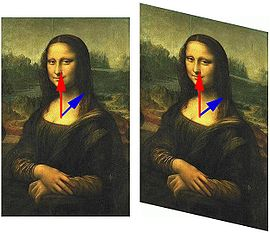#### 求解特征值

eq: 2 »

$det(\lambda \mathbf{I}_n - \underbrace{\mathbf{A}}_{n\times n}) = 0$

\begin{align} det\left( \lambda \begin{bmatrix} 1 & 0 \\ 0 & 1 \end{bmatrix}-\begin{bmatrix} 1 & 2 \\ 4 & 3 \end{bmatrix} \right) &=0 \\ det\left( \begin{bmatrix} \lambda & 0 \\ 0 & \lambda \end{bmatrix}-\begin{bmatrix} 1 & 2 \\ 4 & 3 \end{bmatrix} \right) &=0 \\ det\left( \begin{bmatrix} \lambda -1 & -2 \\ -4 & \lambda -3 \end{bmatrix} \right) &=0 \end{align}

\begin{align} (\lambda -1)(\lambda -3)-8 & =0 \\ \lambda ^{ 2 }-4\lambda -5 &=0 \\ (\lambda - 5)(\lambda +1) &= 0\end{align}

#### 求解特征向量

eq: 3 »

$\mathbf{E}_{\lambda}=N(\lambda I_n - \mathbf{A})$

${ E }_{ \lambda }=N\left( \lambda I_{ n }-\begin{bmatrix} 1 & 2 \\ 4 & 3 \end{bmatrix} \right) =N\left( \lambda \begin{bmatrix} 1 & 0 \\ 0 & 1 \end{bmatrix}-\begin{bmatrix} 1 & 2 \\ 4 & 3 \end{bmatrix} \right) =N\left( \begin{bmatrix} \lambda -1 & -2 \\ -4 & \lambda -3 \end{bmatrix} \right)$

${ E }_{ 5 }=N\left( \begin{bmatrix} 4 & -2 \\ -4 & 2 \end{bmatrix} \right)$

${ E }_{ 5 }= span\left(\begin{bmatrix}\frac{1}{2} \\ 1 \end{bmatrix}\right)$

${ E }_{ -1 }= span\left(\begin{bmatrix}1 \\ -1 \end{bmatrix}\right)$

1. $\mathbb{R}^{n}$ ：表示 n 个有序实数二元组构成的空间。例如 $\mathbb{R}^2$ 表示有序实数二元组 $(x_1, x_2)$ 构成的空间，即$\mathbb{R}^n = \left\{ (x_1, \ldots, x_n) | x_1, \ldots, x_n \in \mathbb{R} \right\}$ 。

2. 从历史的角度讲，该不等式应当称为Cauchy-Buniakowsky-Schwarz不等式【柯西-布尼亚科夫斯基-施瓦茨不等式】，因为，正是后两位数学家彼此独立地在积分学中推而广之，才将这一不等式应用到近乎完善的地步。